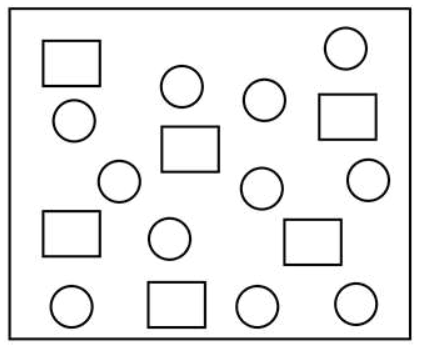# In the figure shown below reactant A (represented by square) is in equilibrium with product B (represented by circle).Question:

In the figure shown below reactant $A$ (represented by square) is in equilibrium with product B (represented by circle). The equilibrium constant is:1. 4

2. 8

3. 1

4. 2

Correct Option: , 4

Solution:

Equilibrium constant

$=\frac{[B]}{[A]} \approx 2$